PetroSkills Blog
By:

# Part-2: Estimation of Solubilities of Benzene, Toluene and Ethylbenzene into Acid Gas Loaded Aqueous MDEA Solutions

Part-2: Estimation of Solubilities of Benzene, Toluene and Ethylbenzene into Acid Gas Loaded Aqueous MDEA Solutions

By:

Mahmood Moshfeghian, Ph.D.

In the Part - 1, July Tip of the month (TOTM), , the following items were discussed briefly:

• Benzene, toluene, ethylbenzene, and xylene are members of the aromatics hydrocarbon family group, often referred to as BTEX. These aromatic compounds are also belonged to the broader category of Hazardous Air Pollutants (HAPs) . BTEX can be present in many natural gas streams and are partially absorbed by the solvent in glycol dehydration and amine (e.g., MDEA) sweetening units. It is of specific interest to note that the components: Benzene, and Ethylbenzene are known carcinogens, thus substantiating their removal be solubility techniques.
•  In gas treating service, methyl diethanolamine (MDEA) will absorb limited quantities of BTEX from the gas. Based on literature data, predicted absorption levels for BTEX components vary from 5 to 30% . Absorption is favored at lower temperatures, higher MDEA concentrations and circulation rates for normal operating Plant Unit Pressures 800 –1200 psia (56 – 84 bar). The bulk of absorbed BTEX is separated from the MDEA in the regeneration unit and leaves the system in the regenerator overhead stream which requires further treatment before being vented to atmosphere.  The amount of absorption of the BTEX is required to be known to determine the proper treating method for the overhead acid gases leaving the regenerator.
• Correctly estimating the quantity of absorbed BTEX and understanding the factors that affect absorption levels is critical to ensure the proper mitigation methods are provided to meet the required emission limits.
• The GPA Midstream research report RR-242 , is an extension of several previous GPA Midstream research projects looking at the solubility of hydrocarbons in loaded and unloaded amine solutions.  The previous research included research reports RR-180, 185, 195, and RR-220.
• Accurate hydrocarbon solubility data of RR-242 enables the development of new equation of state correlations that can be applied to the simulation of amine units (Bullin and Brown [3, 5]). The data can be used to optimize the design and operation of amine units in which these hydrocarbons are present in the feed gas. The data will provide a basis for accurately predicting the distribution of the heavier hydrocarbons between the treated gas, the amine flash gas, and the acid gas streams. The data will provide a basis for accurately predicting emissions of these hydrocarbons from the amine unit to aid in the design and operation of these units (Moshfeghian and Hubbard ).

·       Part – 1 demonstrated applications of the models developed based on the experimental Vapor- Liquid Equilibrium (VLE) and Vapor-Liquid-Liquid Equilibrium (VLLE) data data measurements presented in RR-242 . The models can be used for estimation of solubilities of selected hydrocarbons in pure water, unloaded amine solution, loaded amine solution with CO2 and/or H2S.Figure 1- Process flow diagram of MDEA acid gas recovery unit 

This tip will focus on the following design variables:

• Solubility of selected hydrocarbons in pure water, Equation 1, and Figure 2.
• GPA Midstream RR-242 Proposed Model
• Relative solubility of benzene, toluene, and ethylbenzene (EBenzene), Figure 3.
• Solubility of benzene, toluene, and EBenzene in 50 wt % MDEA solution, Figure 4, and Table 2.
• Multicomponent Hydrocarbons Phase

Solubility of Selected Hydrocarbons in Pure Water:

Solubility of selected hydrocarbons in pure water can be estimated using Equation 1  or Figure 2.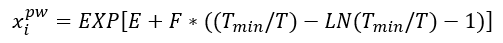(1)

Where T is the temperature in K and the correlation parameters, E, F and Tmin, are shown in Table 1.

Table 1- Equation 1 parameters, E, F and Tmin .Figure 2 was generated using Equation 1 and its parameters in Table 1. The solubility of these selected hydrocarbons in pure water will be used to estimate their solubilities in amine solution in the proceeding sections.Figure 2. Solubility of selected hydrocarbons in pure water .

Estimation of solubility of hydrocarbons in amine solution by the GPA Midstream RR-242 Model 

Equation 2 with its corresponding parameters will be used to estimate the solubility of selected hydrocarbons in unloaded and loaded amine solutions.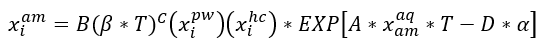(2)

Where:For simplicity and easiness of reading the figures, using the model (Equation 2) similar charts like Figures 3 and 4 or Table 2 were generated in Part – 1 .Figure 3 - Relative solubility of benzene (Fig 3), toluene (Fig 4), and Ethylbenzene (Fig 5) in loaded 50 wt % (13.1 mol-%) MDEA-Water 

Convert 50 wt% MDEA to mole % MDEA

Basis 100 kg of MDEA solution

Mass of MDEA = 50 kg               MW of MDEA = 119.17 kg/kmol

Mass of water = 50 kg                  MW of water = 18 kg/kmol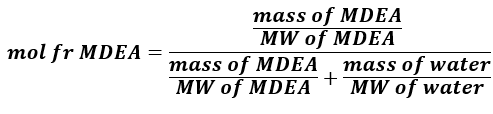Figure 4 - Solubility of benzene, toluene, and ethyl benzene in unloaded 50 wt % MDEA solution 

Table 2 – Solubility (x0) of benzene, toluene, and ethylbenzene in unloaded 50 wt % MDEA solution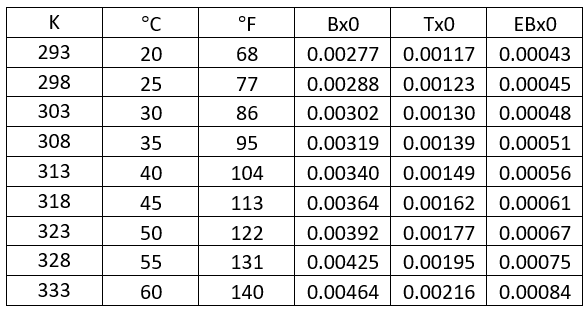Example 1– Single Component Hydrocarbon

This example and its solution on solubility of a single-component benzene in loaded 13.1 mol% (50 wt%) MDEA in water solution were presented in part 1 and shown in Appendix A of this tip.

Multicomponent Hydrocarbons Phase: GPA RR 242  has presented the solubility measurements using a hydrocarbon phase consisting of equimolar amounts of Benzene, Toluene and Ethylbenzene. The acid gas used was CO2. The results are presented in Table 5. To evaluate the accuracy of Eq 2 GPA RR-242 Model , we estimated the solubility of Benzene, Toluene and Ethylbenzene into loaded MDEA (50 wt %) aqueous solution for the conditions listed in Table 5 - GPA RR 242. In addition, a step - by - step hand calculations are shown in Example 2, the yellow highlighted values are the corresponding measured values reported in the highlighted row of Table 5.

Table 5 - Solubility of Benzene, Toluene and Ethylbenzene into loaded MDEA (50 mass-%) aqueous solution Sapphire Apparatus. Hydrocarbon phase consists of x1=0.3329, x2=0.3335, x3=0.3336 [Table 5-GPA RR 242]a is loading mol acid gas/mol amine, T is temperature, P is Pressure, 𝑥̅ – average mole fraction, n - number of analyzed samples, σ - standard deviation

Example 2 – Multicomponent Hydrocarbons Phase

Determine the solubility of benzene, toluene, and ethylbenzene in loaded 13.1 mol% (50 wt%) MDEA in water solution in terms of scf of gas/gal of solvent (std m3 of gas/m3 of solvent) at 60 °C (140 °F), 333 K (600 °R).

The hydrocarbon phase consists of xB = 0.3329, xT = 0.3335, xEB = 0.3336. Rich amine solution acid gas loading,  = 0.41 mol acid gases/ mole MDEA, MDEA MW =119.17

50 wt % MDEA solution density =1017.3 kg/m3 (8.49 lbm/gal)

Solution

From Fig 3 presented in Part 1 (July 2022 TOTM), for  = 0.41 mol acid gases/ mole MDEA,

relative solubility solubilities are, (x/x0)B = 0.52                    (x/x0)T = 0.46                   (x/x0)EB = 0.36

From Table 2 or Fig 4 presented in Part 1, for T =60 °C (140 °F),

the solubilities in unloaded MDEA are, (x0)B = 0.00464       (x0)T = 0.00216                (x0)EB = 0.00084

Specified compositions (mole fractions) of hydrocarbon phase are, xB = 0.3329, xT = 0.3335, xEB = 0.3336Figure 3 - Relative solubility of benzene (Fig 3), toluene (Fig 4), and Ethylbenzene (Fig 5) in loaded 50 wt % (13.1 mol-%) MDEA-Water

Table 2- Solubility of benzene, toluene, and ethyl benzene in unloaded 50 wt % MDEA solution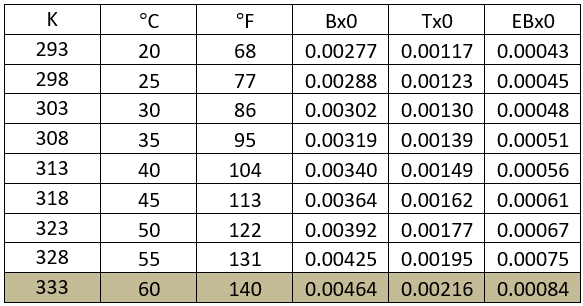Benzene: (x/x0)B = 0.52  (x0)B = 0.00464

For xB = 1, x= x0(x/x0) = (0.00464) (0.52) = 0.00242 kmol Ben/kmol Sol

For xB = 0.3329, x= x0(x/x0) (xB) = (0.00464) (0.52) (0.3329) = 0.0008 (vs. 0.00093) kmol Ben/kmol Sol

The yellow highlighted values are the measured values in Table 5

x = (0.0008 kmol Ben/kmol Sol) (kmol Sol/0.131kmol MDEA) = 0.00611 kmol Ben/kmol MDEA

= (0.00611 kmol Ben/kmol MDEA) (23.64 std m3/kmol Ben) (kmol MDEA/119.21 kg MDEA) =

0.0012 std m3 Ben/kg of MDEA

For each kg of MDEA we need 2 kg of sol (1 kg of MDEA + 1 kg of water)

x = (0.0012 std m3 Ben/kg of MDEA) (kg MDEA/2 kg Sol) (1017.3 kg Sol /m3 Sol)

= 0.62 std m3 of Ben per m3 of solution

Or X = (770) [ (x0) (x/x0) (xB)] std m3 of Ben per m3 of sol

X = (770) [0.0008] = 0.62 std m3 of Ben per m3 of solutionThe same color terms in the numerator and denominator cancel out.

SI CF= (770 std m3 Ben/m3 Sol) (kmol Sol/kmol Ben)

Toluene: (x/x0)T = 0.46                 (x0)T = 0.00216

For xT = 1, x= x0(x/x0) = (0.00216) (0.46) = 0.00099 kmol Tol/kmol MDEA Sol

For xT = 0.3335, x= x0(x/x0) = (0.00099) (0.3335) = 0.00033 vs. 0.00037 kmol Tol/kmol Sol

X = (770) [0.00033] = 0.26 std m3 of Tol per m3 of solution

Ethylbenzene (EB): (x/x0)EB = 0.36           (x0)EB = 0.00084

For xEB = 1, x= x0(x/x0) = (0.00084) (0.36) = 0.00030 kmol EB/kmol MDEA Sol

For xEB = 0.3336, x= x0(x/x0) = (0.00030) (0.3336) = 0.00010 vs. 0.000085 kmol EB/kmol Sol

X = (770) [0.00010] = 0.08 std m3 of EB per m3 of solution

Solution - FPS

Benzene: (x/x0)B = 0.52  (x0)B = 0.00464

For xB = 1, x= x0(x/x0) = (0.00464) (0.52) = 0.00242 lbmol Ben/lbmol MDEA Sol

For xB = 0.3329, x= x0(x/x0) = (0.00464) (0.52) (0.3329) = 0.0008 (vs. 0.00093) lbmol Ben/lbmol Sol

The yellow highlighted values are the measured values in Table 5

x = (0.0008 lbmol Ben/lbmol Sol) (lbmol Sol/0.131lbmol MDEA) = 0.00611 lbmol Ben/lbmol MDEA

x = (0.00611 lbmol Ben/lbmol MDEA) (379.5 scf/lbmol Ben) (lbmol MDEA/119.21 lbm)

= 0.01945 scf Ben/lbm of MDEA

For each lbm of MDEA we need 2 lbm of sol (1 lbm of MDEA + 1 lbm of water)

x = (0.01945 scf Ben/lbm of MDEA) (lbm MDEA/2 lbm Sol) (8.49 lbm/gallon Sol)

= 0.083 scf of Ben/gallon solution

Or X = (103) [(x0) (x/x0) (xB)] scf of Ben/gallon of solution

X = (103) [0.0008] = 0.082 scf of Ben/gallon of solutionThe same color terms in the numerator and denominator cancel out.

FPS CF = (103 scf Ben/gallon Sol) (lbmol Sol/lbmol Ben)

Toluene: (x/x0)T = 0.46                 (x0)T = 0.00216

For xT = 1, x= x0(x/x0) = (0.00216) (0.46) = 0.00099 kmol Tol/kmol Sol

For xT = 0.3335, x= x0(x/x0) = (0.00099) (0.3335) = 0.00033 vs. 0.00037 kmol Tol/kmol Sol

Or X = (FPS CF) [(x0) (x/x0) (xT)] scf of Ben/gallon of solution

X = (103) [0.00033] = 0.034 scf of Ben/gallon of solution

Ethylbenzene (EB): (x/x0)EB = 0.36           (x0)EB = 0.00084

For xEB = 1, x= x0(x/x0) = (0.00084) (0.36) = 0.00030 kmol EB/kmol MDEA Sol

For xEB = 0.3336, x= x0(x/x0) = (0.00030) (0.3336) = 0.00010 vs. 0.000085 kmol EB/kmol Sol

Or X = (103) [(x0) (x/x0) (xEB)] scf of Ben/gallon of solution

X = (103) [0.00010] = 0.010 scf of Ben/gallon of solution

The estimated soluble BTE in the MDEA solution for example 2 and two other cases are compared with the measured values in Table 5R. This table indicates that the APD (absolute percent difference between the measured and estimated values) for eight out of nine estimated solubilities are less than 17.2. Considering very small amount of solubility, the Model estimation values are good facilities calculations and troubleshooting.

Table 5R – Measured and estimated solubility of Benzene, Toluene and Ethylbenzene into loaded MDEA (50 wt %) aqueous solution. Hydrocarbon phase consists of xB=0.3329, xT=0.3335, xEB=0.3336APD = Absolute percent deviation =100*ABS (Exp Avg x – Model x) / (Exp Avg x)

Summary

From the RR-242 developed model based on the experimental results; several conclusions can be drawn.

• Figures 3 and 4 or Table 2 present a simple tool for quick estimation of solubility of BETX compounds in 50 wt % MDEA gas treating process.
• Figures 3 and 4 or Table 2 are suitable for 50 wt % (13.1 mol-%) MDEA in the MDEA-Water solution, similar chart and tables can generated for other different amines and concentrations.
• For the examples considered in this tip and July TOTM, the estimated solubility for each of BETX compounds matched well with the experimental results.

To learn more about similar cases and how to minimize operational problems, we suggest attending our G4 (Gas Conditioning and Processing), G5 (Advanced Applications in Gas Processing), http://www.jmcampbell.com/co2-surface-facilities-pf81.php and PF49 (Troubleshooting Oil & Gas Processing Facilities), courses.

By: Mahmood Moshfeghian, Ph.D.

References:

1. Moshfeghian, M. and Snow–McGregor, K., www.petroskills.com/en/blog/entry/july2022-totm, PetroSkills July TOTM, 2022.

2. http://www.earthworksaction.org/BTEX.cfm, 2011.

3. Campbell, J. M. “Gas conditioning and processing, Volume 2: The Equipment Modules,” 9th Edition, 2nd Printing, Editors Hubbard, R. and Snow–McGregor, K., Campbell Petroleum Series, Norman, Oklahoma, 2014.

4. Uusi-Kyyny, P., Pakkanen, M., Richon, D., Ionita, S., Ogunrobo, E., Alopaeus, V., RR-242, “Solubility of Hydrocarbons in Amine Treating Solutions”, GPA Midstream Association, Tulsa, OK, 2019.

5. Bullin, J. A., Brown, W.G., Hydrocarbons and BTEX Pickup and Control from Amine Systems”, 83rd  Gas Processors Association Annual Convention, Mar. 2004.

6. Moshfeghian, M. and R.A. Hubbard, “Quick Estimation of Absorption of Aromatics Compounds (BTEX) in TEG Dehydration Process”, 3rd International Gas Processing Symposium, March 5-7, Doha, Qatar, 2012.

Appndix A

Example 1

Determine the solubility of benzene in loaded 13.1 mol% (50 wt%) MDEA in water solution in terms of scf of gas/gal of solvent (std m3 of gas/m3 of solvent) at 60 °C (140 °F), 333 K (600 °R).

Rich amine solution acid gas loading,  = 0.4 mol acid gases/ mole MDEA, MDEA MW =119.17

50 wt % MDEA solution density =1017.3 kg/m3 (8.49 lbm/gal)

Solution

From Fig 1, for  = 0.4 mol acid gases/ mole MDEA, relative solubility, x/x0= 0.53

Benzene solubility in un-loaded MDEA, x0 = 0.00464Figure 1 - Relative solubility of Benzene in 50 weight % (13.1 mol-%) MDEA-Water [Figure 16 of GPA RR 242].

Solution - SI

X = x0(x/x0) = (0.00464) (0.53kmol Ben/kmol MDEA Sol) (kmol MDEA Sol/0.131kmol MDEA)

= (0.0187 kmol Ben/kmol MDEA) (23.64 std m3/kmol Ben) (kmol MDEA/119.21 kg)

= (0.0037 std m3 Ben/kg of MDEA) (kg MDEA/2 kg MDEA + Water Sol) (1017.3 kg/m3 MDEA Sol)

= 1.88 std m3 of Ben/m3 of MDEA + water solution

Or X = (770) (x0) (x/x0) std m3 of Ben/m3 of MDEA + water solution

Solution - FPS

X = x0(x/x0) = (0.00464) (0.53 lbmol Ben/lbmol MDEA Sol) (lbmol MDEA Sol/0.131lbmol MDEA)

= (0.0187 lbmol Ben/lbmol MDEA) (379.5 scf/lbmol Ben) (lbmol MDEA/119.21 lbm)

= (0.0598 scf Ben/lbm of MDEA) (lbm MDEA/2 lbm MDEA + Water Sol) (8.49 lbm/gallon MDEA Sol)

= 0.25 scf of Ben/gallon of MDEA + water solution

Or X = (103) (x0) (x/x0) scf of Ben/gallon of MDEA + water solution

## Sidebar

### Featured posts###### Hydrogen Series Part 1: Hydrogen Colors – What do they mean, Options for Production and their associated Challenges?
by Kindra Snow-McGregor

### Popular tags

#Renewables #LNG #Unconventionals